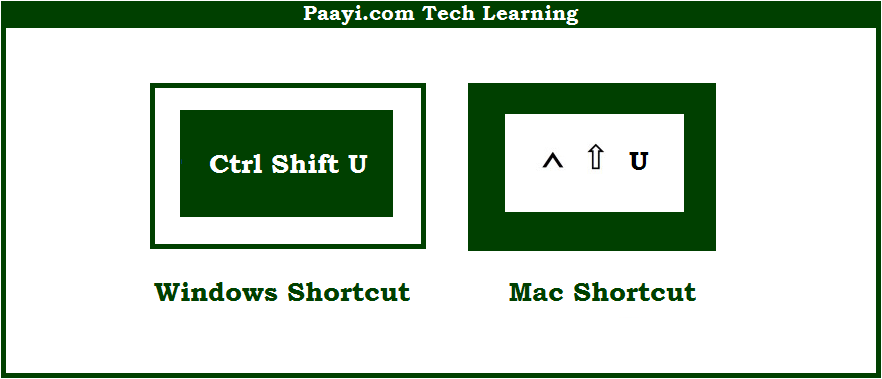# Learn How to Expand or Collapse the Formula Bar With Shortcut in Microsoft Excel

Written by | 0 Comments | 606 Views

In this article, you will learn how to Expand or Collapse the Formula Bar with a shortcut in Microsoft Excel. You will also get to know the shortcut to Expand or Collapse the Formula Bar on Mac.

Expand or Collapse the Formula Bar With Shortcut in Microsoft Excel

Here we will show you how to expand or collapse the formula bar in Microsoft Excel. Like many other programs Microsoft Excel also uses similar key combinations while using shortcuts.

You can use the key combinations given below to expand or collapse the formula bar using the shortcut. This keyboard shortcut is used to toggle the formula bar between a collapsed and expanded state. It is especially useful when you are having a very long formula, or have added line breaks to a formula for readability. So, here we go:

## How to Expand or Collapse the Formula Bar With Shortcut in Microsoft Excel?It is used to expand or collapse the formula bar in Microsoft Excel.  This keyboard shortcut is used to toggle the formula bar between a collapsed and expanded state. It is especially useful when you are having a very long formula, or have added line breaks to a formula for readability. This way you can easily expand or collapse the formula bar using this shortcut.